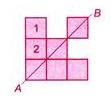Courses

# Test : Symmetry - 1

## 10 Questions MCQ Test Mathematics for Class 5 (V) - CBSE and NCERT Curriculum | Test : Symmetry - 1

Description
This mock test of Test : Symmetry - 1 for Class 5 helps you for every Class 5 entrance exam. This contains 10 Multiple Choice Questions for Class 5 Test : Symmetry - 1 (mcq) to study with solutions a complete question bank. The solved questions answers in this Test : Symmetry - 1 quiz give you a good mix of easy questions and tough questions. Class 5 students definitely take this Test : Symmetry - 1 exercise for a better result in the exam. You can find other Test : Symmetry - 1 extra questions, long questions & short questions for Class 5 on EduRev as well by searching above.
QUESTION: 1

### How many of the following figures have no line of symmetry?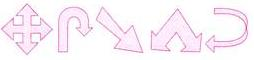Solution: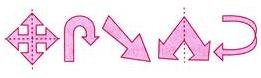So, 3 figures have no line of symmetry.

QUESTION: 2

### What is the least number of squares that must be added so that the line MN becomes a line of symmetry?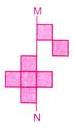Solution: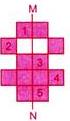QUESTION: 3

### How many more squares in the figure must be drawn so that the figure becomes symmetrical along the dotted line?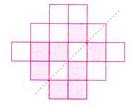Solution: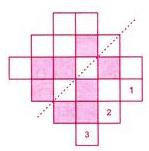QUESTION: 4

Which of the following is a line of symmetry of the figure?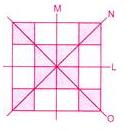Solution:
QUESTION: 5

What is the smallest number of squares that must be shaded so that the figure has PQ as line of symmetry?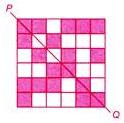Solution: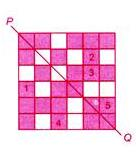QUESTION: 6

Which of the following figures has a line of symmetry?

Solution:
QUESTION: 7

Which of the following figures has a Sine of symmetry?

Solution: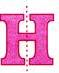QUESTION: 8

Which of the following figure(s) does/do not have a line of symmetry?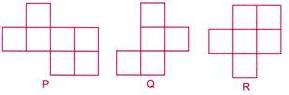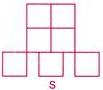Solution: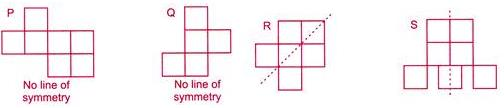QUESTION: 9

Which of the following figures does not have a line of symmetry?

Solution: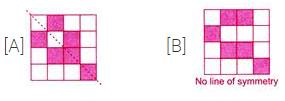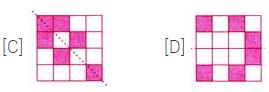QUESTION: 10

What is the least number of squares that must be added, so that the line AB becomes a line of symmetry?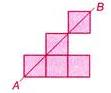Solution: